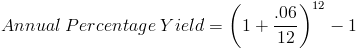# Annual Percentage YieldThe Annual Percentage Yield (APY), referenced as the effective annual rate in finance, is the rate of interest that is earned when taking into consideration the effect of compounding.

There are various terms used when compounding is not considered including nominal interest rate, stated annual interest rate, and annual percentage rate(APR).

In the formula, the stated interest rate is shown as r. A bank may show this as simply "interest rate". The annual percentage yield formula would be applied to determine what the effective yield would be if the account was compounded given the stated rate. The n in the annual percentage yield formula would be the number of times that the financial institution compounds. For example, if a financial institution compounds the account monthly, n would equal 12.

## Example of Annual Percentage Yield

An account states that its rate is 6% compounded monthly. The rate, or r, would be .06, and the number of times compounded would be 12 as there are 12 months in a year. When we put this into the formula we haveAfter simplifying, the annual percentage yield is shown as 6.168%.

New to Finance?